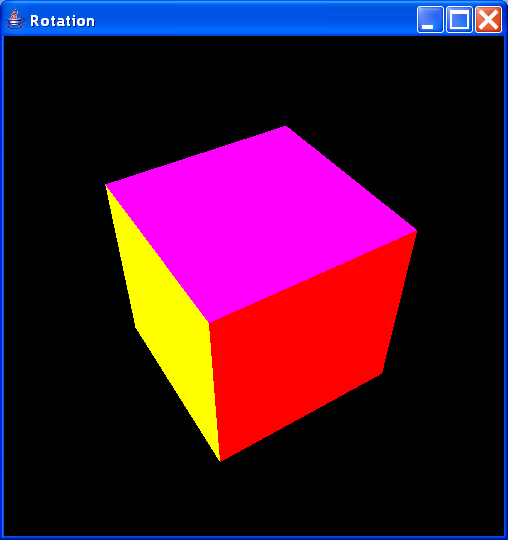# Matrix « 2D Graphics GUI « Java

1.Implementation of a 4x4 matrix suited for use in a 2D and 3D graphics rendering engine
2.Rotations in a three-dimensional space3.This class represents a lower (or upper) triangle matrix that stores ints.
4.The Java Matrix Class provides the fundamental operations of numerical linear algebra
5.Vector extends Matrix
6.A 3x3 matrix implementation
7.4 x 4 Matrix
8.Various geometric transformations on matrix form
9.Inertia Matrix
10.Simulate a matrix. Provides method to travers vectors that compose the matrix.